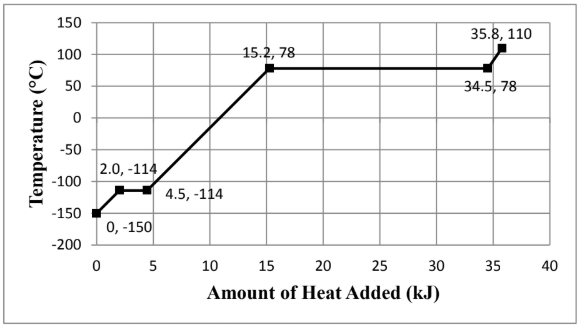# Problem: Consider the heating curve shown below for 22.86 grams of an unknown substance. You may assume constant pressure conditions. Note: the points are labeled as (amount of heat added, temperature). Calculate the heat capacity at constant pressure, C p, (in J/mol∙K) for the gas. Show all work and circle your final answer. The moles were identified to be 0.496 mol.

###### FREE Expert Solution

We are asked to heat capacity at constant pressure, C p, (in J/mol∙K) for the gas

We go through the following steps to solve this problem:

Step 1. Calculate the amount of heat added based on the heating curve

Step 2. Calculate the heat capacity

96% (150 ratings)###### Problem Details

Consider the heating curve shown below for 22.86 grams of an unknown substance. You may assume constant pressure conditions. Note: the points are labeled as (amount of heat added, temperature).Calculate the heat capacity at constant pressure, C p, (in J/mol∙K) for the gas. Show all work and circle your final answer. The moles were identified to be 0.496 mol.

Frequently Asked Questions

What scientific concept do you need to know in order to solve this problem?

Our tutors have indicated that to solve this problem you will need to apply the Heating and Cooling Curves concept. You can view video lessons to learn Heating and Cooling Curves. Or if you need more Heating and Cooling Curves practice, you can also practice Heating and Cooling Curves practice problems.